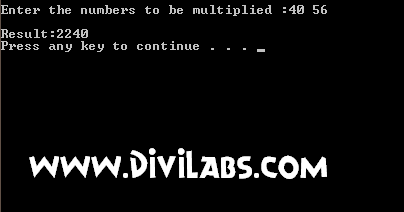# C / C++ Program to Multiply two Numbers using BITWISE operations

/* The following C / C++ code will be requiring two numbers from user as input. Then the program will perform bitwise AND till the second number doesn't become '0', first value is left shifted & 2nd value is right shifted in each iteration. Left Shifting multiplies by 2 for each loop where as Right Shifting divides by 2 for each loop*/
//CODE:-
#include<stdio.h>
#include<stdlib.h>
void main()
{
int a,b,res;
printf("\nEnter the numbers:");

scanf("%d%d",&a,&b); // Assuming you entered a>b
res=0;
while(b != 0)
// Iterate the loop till b==0
{
if (b&01)
// Bitwise & of the value of b with 01
{
res=res+a;
// Add a to result if b is odd .
}
a<<=1;
// Left shifting the value contained in 'a' by 1
// multiplies a by 2 for each loop
b>>=1; // Right shifting the value contained in 'b' by 1.
}
printf("\nResult:%d\n",res);
system("pause");
}

Screenshot:C / C++ Program to Multiply two Numbers using BITWISE operations Output